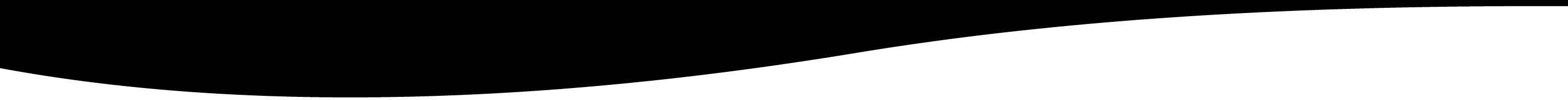# tutorial_sncode_variables

This manual page describe variables in Sncode

# Tutorial-Variables

## NAME

Variables- This manual page describe variables in Sncode

## DESCRIPTION

Variables in Sncode start with a letter in lowercase or in uppercase or a _ followed by letters, numbers or _.

For example:

```a = 5;
b0 = "6";```

Variables in Sncode do not have to be declared.

The type of the variable is defined by the type on the left side of the assigment.

So :

```a = 5;        // a is an integer
b0 = "6";     // b0 is a string```

To print the value of the variable, just specify the name of the variable followed by ';' as in:

```a = 5;        // a is an integer
b0 = "6";     // b0 is a string
a;            // Display the value of a
```

If you want to have more control on the format of the output, you can use function printf() which we will see later on.

The following types are supported by Sncode:

integer
An integer (from the Latin integer meaning "whole") is a number that can be written without a fractional component. For example, 21, 4, 0, and −2048 are integers, while 9.75, 5½, and √2 are not.

The set of integers consists of zero (0), the natural numbers (1, 2, 3, ...), also called whole numbers or counting numbers, and their additive inverses (the negative integers, i.e. −1, −2, −3, ...).

float
Representent a real number. In mathematics, a real number is a value that represents a quantity along a continuous line.

The real numbers include all the rational numbers, such as the integer −5 and the fraction 4/3, and all the irrational numbers such as √2 (1.41421356…, the square root of two, an irrational algebraic number) and π (3.14159265…, a transcendental number).

The internal representation of a float is like a double in the C programming language.

string
A string is a sequence of character implemented as an array. To represent a the string value, you have to enclosed the string in quotes.

Quote can be 'abc\n'. The sequence \n is output as \ followed by n.

Quote can be "abc\n". The sequence \n is output as character newline. In a double quote string the following sequence are output as the corresponding value:

\n
\\
\a
\b
\v
\f
\t
\r
\xab output the character with the corresponing hexadecimal value. For example \x65 output character e.
\u for utf-8 character. As an example: "\ue79a85"; output 皅
\o for octal number

Quote can also be q?abc\n?. Use a different quote character.

Quote can also be qq?abc\n?. Use a differente quote character. \n is output as a newline.

Quote can also be dq{[abc\n]}. Use two characters for quoting. (), {}, [], <> or the same character. \n is output as \ followed by n.

Quote can also be dqq{[abc\n]}. Use two characters for quoting. (), {}, [], <> or the same character. \n is output as a newline.

bool
A bool is a variable that can take two values: true or false.
Example:
`a = true;`

array
An array is a sequence of items. The items can be any types. An array is predefined with a certain length and the length will be increased if needed.
The array is never shrinked. A new array can be create using the function array().
Example:

`a = [ 1, 2.1, true, "abc", null, undefined, { "x" : 1 }, [1, 2, 3] ]; a = array(10);`

associative array
An associative array, map, symbol table, or dictionary is an abstract data type composed of a collection of [(key, value)] pairs, such that each possible key appears just once in the collection.
Example:
`a = { "x" : 2, "y" : 3 };`

The key part of the definition array can be a variable which will be replace by its value for the key as in:
```    x = "laplante";
a = { x : 1, "x" : 2, "y" : 3 };

for i in a do
"i="; i;
endfor```

null, undefined, pointer
An integer (from the Latin integer meaning "whole") is a number that can be written without a fractional component. For example, 21, 4, 0, and −2048 are integers, while 9.75, 5½, and √2 are not.

The set of integers consists of zero (0), the natural numbers (1, 2, 3, ...), also called whole numbers or counting numbers, and their additive inverses (the negative integers, i.e. −1, −2, −3, ...).

## EXERCICES

• Will this work: é = 5; é; ?
• and #4 = 5; ?
• Do you think a=5; and A=5; are the same ?
• If we say a=5; a="5"; a.type(); what will be the type of a?